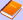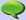ContentsElectronics TheoryElecttronics SchematicsMathComputerLetterature and ArtQuotes

 PhotosGalliate's WebcamThe best photosPlaces and landscapesPlantsAnimalsVariousPanoramasHDRNewsLinksBlog05 - While and until cycles in the bash

 Previous page | Next page

The while..do..done construct executes a group of operations while a condition is valid. The syntax is:

```while [condition]; do   code1 done```

Two numbers can be compared with the following tests. The variables must be written in the form:

`"\$variable_name"`

The numerical tests are:

 Control Meaning x -eq y true if x is equal to y x -ne y true if x is not equal to y x -gt y true if x is bigger than y x -lt y true if x is smaller than y x -ge y true if x is bigger than or equal to y x -le y true if x is smaller than or equal to y

Two strings can be compared with the following tests:

 Control Meaning x = y true if x is equal to y x != y true if x is equal to y -n x true if x is not NULL or void -z x true if x is NULL or void

Here is an example script:

```#!/bin/bash # The following script increments a variable # up to 10, printing each time the result x=0 while [ "\$x" -le 10 ]; do   echo "Valore corrente di x: \$x";   x=\$(( \$x + 1 )) done  ```

A construct similar to while is until, that tests the condition on the opposite way: executes some code while a condition is false (that is, until a condition becomes true). Here is an example:

```#!/bin/bash # The followinf example intrements a variable up # to 10, printing each time the result x=0 until [ "\$x" -ge 10 ]; do   echo "Current x value: \$x";   x=\$(( \$x + 1 )) done  ```

 Previous page | Next page

Fatal error: Call to undefined function sqlite_open() in /membri/giacobbe85/include/commenti.inc.php on line 324